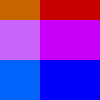# get_np_pixels()#

Get the contents of np_pixels[] as a numpy array.

## Examples#def setup():
py5.background(0)
array = py5.get_np_pixels(bands='RGB')
array[:60, :, 0] = 200
array[:, :40, 1] = 100
array[20:, :, 2] = 250
py5.set_np_pixels(array, bands='RGB')def setup():
py5.background(32)
array = py5.get_np_pixels(10, 10, 80, 80, bands='RGB')
array[:60, :, 0] = 200
array[:, :40, 1] = 100
array[20:, :, 2] = 250

py5.np_pixels[10:90, 10:90, 1:] = array
py5.update_np_pixels()


## Description#

Get the contents of np_pixels[] as a numpy array. The returned numpy array can be the entirety of np_pixels[] or a rectangular subsection. Use the x, y, h, and w parameters to specify the bounds of a rectangular subsection.

The bands parameter is used to determine the ordering of the returned numpy array’s color channel. It can be one of 'L' (single-channel grayscale), 'ARGB', 'RGB', or 'RGBA'. If the bands parameter is 'L', the returned array will have two dimensions, and each pixel value will be calculated as 0.299 * red + 0.587 * green + 0.114 * blue. The alpha channel will also be ignored. For all other bands parameter values, the returned array will have three dimensions, with the third dimension representing the different color channels specified by the bands value.

The returned array will always be a copy of the data in np_pixels[] and not a view into that array or any other array. Use the dst parameter to provide the numpy array to copy the pixel data into. The provided array must be sized correctly. The array’s dtype should np.uint8, but this isn’t required.

## Signatures#

get_np_pixels(
*,
bands: str = "ARGB",  # color channels in output array
dst: npt.NDArray[np.uint8] = None  # destination array to copy pixel data into
) -> npt.NDArray[np.uint8]

get_np_pixels(
x: int,  # x-coordinate of the source's upper left corner
y: int,  # y-coordinate of the source's upper left corner
w: int,  # source width
h: int,  # source height
/,
*,
bands: str = "ARGB",  # color channels in output array
dst: npt.NDArray[np.uint8] = None,  # destination array to copy pixel data into
) -> npt.NDArray[np.uint8]


Updated on August 07, 2023 14:29:21pm UTC